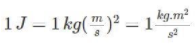Home   »   What is Unit Of Energy in...

# What is Unit Of Energy in SI, MKS, CGS

## What is Unit Of Energy in SI, MKS, CGS?

Unit of Energy is defined as the capacity of doing work. There are various forms of energy like kinetic, potential, electrical, chemical, nuclear, thermal, and other forms. Energy can  be classifies in two types i.e. Renewable energy and Non-renewable energy. Renewable energy is generated from natural sources and is naturally replenished on a human timescale. It includes sources such as sunlight, wind, rain, tides, waves, and geothermal heat. Non renewable energy is that energy that cannot be readily replaced by natural means at a pace quick enough to keep up with consumption. An example is carbon-based fossil fuels.

## What is the SI Unit of Energy?

The SI unit of Energy is Joules. Energy is a scalar quantity. It is named after a British physicist, James Prescott Joule. He contributions in understanding the concept of energy is very important.  When we look at the unit in fundamental terms, 1-N.m is equal to 1 Joule.## What is the CGS Unit of Energy?

The CGS Unit of Energy is erg. The erg is a unit of energy equal to 10⁻⁷ . An erg can be defined as the amount of the work done by the force of one dyne on one-centimeter distance.

## What is MKS Unit of Energy?

The MKS Unit of Energy is Joule.

## Commercial Unit of Energy

The commercial unit of energy is the kilowatt-hour. When huge amounts of energy  are expressed, the SI unit of energy becomes small. As a result, the commercial unit is employed.

## List of Energy Units

Energy units can be preceded by a variety of elements, and different units are typically used to measure energy. The following are some examples of common units:

1. British thermal unit (BTU)
2. Horsepower
3. Kilowatt-hour (kWh)
4. Calorie
5. Electronvolts (eV)
6. Hartree (the atomic unit of energy)
7. Rydberg units
8. Barrel of oil

## Conversion of Energy

Below is the complete list given if energy conversions.

 Unit Joule Calorie BTU Kilowatt-hour Joule 00 0.2390 0.000948 2.77778E-07 Calorie 4.187 00 0.00397 1.16279E-06 BTU 1055 252 00 0.000293 Kilowatt-hour 3.6E6 8.6E5 3412 00

## Frequently Asked Questions

Q. What’s the difference between a commercial and a SI energy unit?

Ans. The commercial unit of energy is the kilowatt-hour (kWh). The quantity of energy utilised by a device in one working hour at a constant rate of one kilowatt is defined as one kilowatt-hour.

The SI unit of energy is Joule. As a result, the following is the relationship between the commercial and SI units of energy:

1 kWh = 1kW x 1h = 1000W x 1h = 1000(J/s) x 3600 s = 3.6 x106 J

Q. What’s the connection between energy and power?

Ans. Power and energy are related in that power has units of energy that are split by time. Watts is the unit of power.

• Joules are a unit of energy.
• 1 Joule/1 second Equals 1 WattThank You, Your details have been submitted we will get back to you.